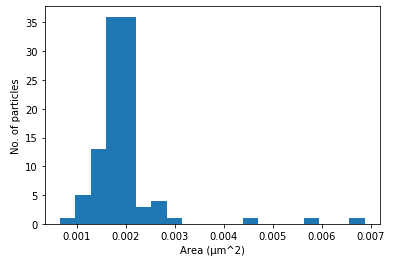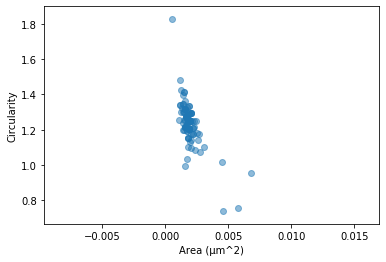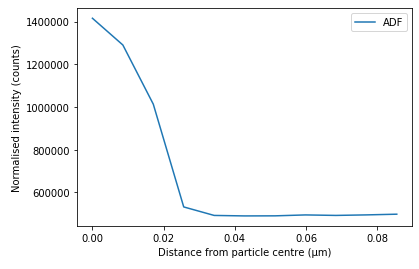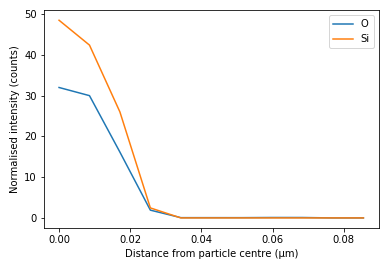# Plotting and Saving¶

One of the main benefits of ParticleSpy is the ability to plot particle properties natively. The main method for plotting particle properties is `plot()`. This method plots either a histogram of any specified property or a scatter plot of two properties, as long as you have added these properties to each particle (either automatically or manually).

An example of plotting a histogram of particle areas in shown here:

```>>> particles = ps.particle_analysis(data, params, mask=generated_mask)
>>> particles.plot('area', bins = 20)
```In the above code it is possible to plot particle area because this is automatically calculated in the particle_analysis function.

To plot a scatter plot of two properties you simply need to specify two properties in the arguments:

```>>> particles.plot(['area','circularity'])
```It is also possible to plot a 3D scatter plot of three properties by specifying three properties in the argument.

Plotting of more than one particle_list can be done using the top level `plot()` function:

```>>> ps.plot([particles1,particles2],['area','circularity'])
```

All keyword arguments in matplotlib are available by passing them as arguments to the corresponding plotting function.

ParticleSpy provides the ability to plot a radial profile (that is an intensity profile from particle centre to edge) of image intensity or EDS signal intensity. A radial profile can be very useful for illustrating distributions in particles.

The following code shows how to plot a radial profile of image intensity.

```>>> rp = ps.radial_profile(particle,['Image'],plot=True)
```It is also possible to plot the intensity of certain elements from an EDS signal.

```>>> rp = ps.radial_profile(particle,['Pt','Ni'],plot=True)
```## Saving Particle Images and Maps¶

In order to save images and maps of particles it is necessary to use Hyperspy’s save function.

```>>> particles.list.image.save(filename)
```